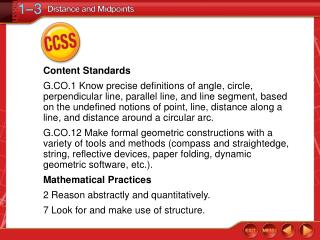Download PresentationCCSS

# CCSS

Download Presentation## CCSS

- - - - - - - - - - - - - - - - - - - - - - - - - - - E N D - - - - - - - - - - - - - - - - - - - - - - - - - - -
##### Presentation Transcript

1. Content Standards G.CO.1 Know precise definitions of angle, circle, perpendicular line, parallel line, and line segment, based on the undefined notions of point, line, distance along a line, and distance around a circular arc. G.CO.12 Make formal geometric constructions with a variety of tools and methods (compass and straightedge, string, reflective devices, paper folding, dynamic geometric software, etc.). Mathematical Practices 2 Reason abstractly and quantitatively. 7 Look for and make use of structure. CCSS

2. You graphed points on the coordinate plane. • Find the distance between two points. • Find the midpoint of a segment. Then/Now

3. distance • irrational number • midpoint • segment bisector Vocabulary

4. Concept

5. Find Distance on a Number Line Use the number line to find QR. The coordinates of Q and R are –6 and –3. QR = | –6 – (–3) | Distance Formula = | –3 | or 3 Simplify. Answer: 3 Example 1

6. Use the number line to find AX. Example 1

7. Switching both coordinates in the formula will give the same result for the distance. See below. Concept

8. (x1, y1) = (–4, 1) and (x2, y2) = (3, –1) Find Distance on a Coordinate Plane Find the distance between E(–4, 1) and F(3, –1). Example 2

9. Find Distance on a Coordinate Plane CheckGraph the ordered pairs and check by using the Pythagorean Theorem. Draw a sketch of this graph on your paper. Example 2

10. . Find Distance on a Coordinate Plane Example 2

11. Find the distance between A(–3, 4) and M(1, 2) using the distance formula. Example 2

12. Chapter 1 Overview Video Distance and Midpoint Skills Packet Do 7 and 8 (find the distance only) Now let’s do the Reach test questions 1-3

13. Concept

14. Concept

15. Find Midpoint in Coordinate Plane Answer:M(–3, 3) Example 4

16. Class Assignment - 8 minutes Distance and Midpoint Skills Packet Do 4, 5 and 8 (Find the midpoint only)

17. (x2, y2) = (–5, –3) Find the Coordinates of an Endpoint Let D be (x1, y1) and F be (x2, y2) in the Midpoint Formula. Write two equations to find the coordinates of D. Example 5

18. Midpoint Formula Midpoint Formula Find the Coordinates of an Endpoint Answer: The coordinates of D are (–7, 11). Example 5

19. 5 minutes Skills Packet Do 9 and 10

20. UnderstandYou know that Q is the midpoint of PR, and the figure gives algebraic measures for QR and PR. You are asked to find the measure of PR. Use Algebra to Find Measures Example 6

21. PlanBecause Q is the midpoint, you know that Subtract 1 from each side. Use Algebra to Find Measures Use this equation and the algebraic measures to find a value for x. Solve Example 6

22. Original measure Use Algebra to Find Measures Example 6

23. Example 6

24. Constructions p. 30 ‘Bisect a Segment

25. HW Skills Packet 1-7, 12-16 all